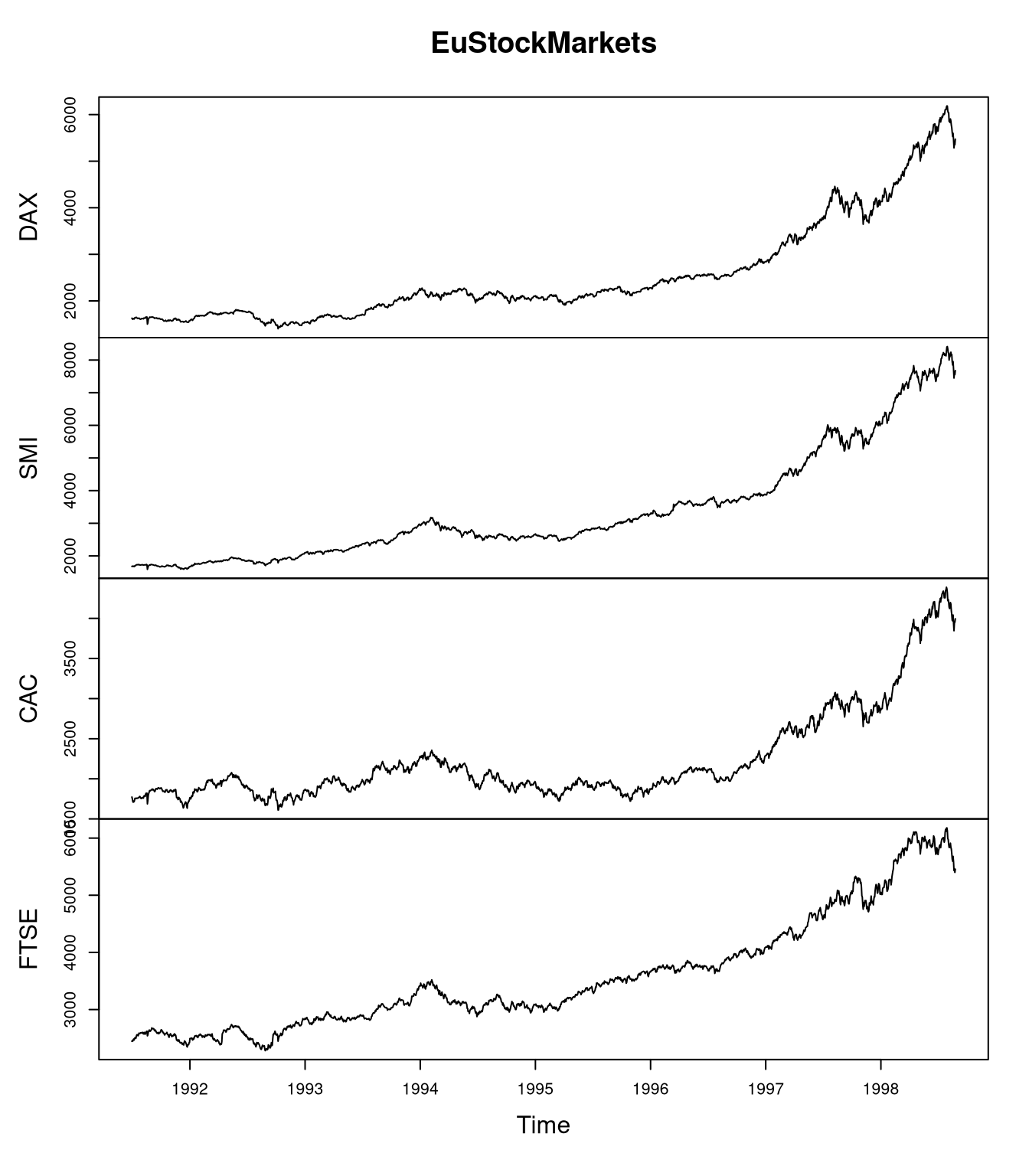[This article was first published on R on datascienceblog.net: R for Data Science, and kindly contributed to R-bloggers]. (You can report issue about the content on this page here)
Want to share your content on R-bloggers? click here if you have a blog, or here if you don't.

The line plot is the go-to plot for visualizing time-series data (i.e. measurements for several points in time) as it allows for showing trends along time. Here, we’ll use stock market data to show how line plots can be created using native R, the MTS package, and ggplot.

## The EuStockMarkets data set

The EuStockMarkets data set contains the daily closing prices (except for weekends/holidays) of four European stock exchanges: the DAX (Germany), the SMI (Switzerland), the CAC (France), and the FTSE (UK). An important characteristic of these data is that they represent stock market points, which have different interpretations depending on the exchange. Thus, one should not compare points between different exchanges.

```data(EuStockMarkets)
summary(EuStockMarkets)
##       DAX            SMI            CAC            FTSE
##  Min.   :1402   Min.   :1587   Min.   :1611   Min.   :2281
##  1st Qu.:1744   1st Qu.:2166   1st Qu.:1875   1st Qu.:2843
##  Median :2141   Median :2796   Median :1992   Median :3247
##  Mean   :2531   Mean   :3376   Mean   :2228   Mean   :3566
##  3rd Qu.:2722   3rd Qu.:3812   3rd Qu.:2274   3rd Qu.:3994
##  Max.   :6186   Max.   :8412   Max.   :4388   Max.   :6179
class(EuStockMarkets)
##  "mts"    "ts"     "matrix"```

What is interesting is that the data set is not only a matrix but also an mts and ts object, which indicate that this is a time series object.

In the following, I will show how these data can be plotted with native R, the MTS package, and, finally, ggplot.

## Creating a line plot in native R

Creating line plots in native R is a bit messy because the `lines` function does not create a new plot by itself.

```# create a plot with 4 rows and 1 column
par(mfrow=c(4,1))
# set x-axis to number of measurements
x <- seq_len(nrow(EuStockMarkets))
for (i in seq_len(ncol(EuStockMarkets))) {
# plot stock exchange points
y <- EuStockMarkets[,i]
# show stock exchange name as heading
# create empty plot as template, don't show x-axis
plot(x, y, type="n", main = heading, xaxt = "n")
# add actual data to the plot
lines(x, EuStockMarkets[,i])
# adjust x tick labels to years
years <- as.integer(time(EuStockMarkets))
tick.posis <- seq(10, length(years), by = 100)
axis(1, at = tick.posis, las = 2, labels = years[tick.posis])
}```The plot shows us that all of the European stock exchanges are highly correlated and we could use the plot to explain the stock market variation based on past economic events.

Note that this is a quick and dirty way of creating the plot because it assumes that the time between all measurements is identical. This approximation is acceptable for this data set because there are (nearly) daily measurements. However, if there were time periods with lower sampling frequency, this should be shown by scaling the axis according to the dates of the measured (see the ggplot example below).

## Creating a line plot with the MTS package

If you have an object of type mts, then it is much easier to use the plot function from the MTS package. It gives a similar but admittedly more beautiful plot than the one I manually created using native R above.

`plot(EuStockMarkets)`## Creating a line plot with ggplot

To create the same plot with ggplot, we need to construct a data frame first. In this example, we want to consider the dates at which the measurements were taken when scaling the x-axis.

The problem here is that the mts object doesn’t store the years as dates but as floating point numbers. For example, a value of 1998.0 indicates a day in the beginning of 1998, while 1998.9 indicates a value at the end if 1998. Since I could not find a function that transforms such representations, we will create a function that transforms this numeric representation to dates.

```scale.value.range <- function(x, old, new) {
# scale value from interval (min/max) 'old' to 'new'
scale <- (x - old) / (old - old)
newscale <- new - new
res <- scale * newscale + new
return(res)
}
float.to.date <- function(x) {
# convert a float 'x' (e.g. 1998.1) to its Date representation
year <- as.integer(x)
# obtaining the month: consider decimals
float.val <- x - year
# months: transform from [0,1) value range to [1,12] value range
mon.float <- scale.value.range(float.val, c(0,1), c(1,12))
mon <- as.integer(mon.float)
date <- get.date(year, mon.float, mon)
return(date)
}
days.in.month <- function(year, mon) {
# day: transform based on specific month and year (leap years!)
date1 <- as.Date(paste(year, mon, 1, sep = "-"))
date2 <- as.Date(paste(year, mon+1, 1, sep = "-"))
days <- difftime(date2, date1)
return(as.numeric(days))
}
get.date <- function(year, mon.float, mon) {
max.nbr.days <- days.in.month(year, mon)
day.float <- sapply(seq_along(year), function(x)
scale.value.range(mon.float[x] - mon[x], c(0,1), c(1,max.nbr.days[x])))
day <- as.integer(day.float)
date.rep <- paste(as.character(year), as.character(mon),
as.character(day), sep = "-")
date <- as.Date(date.rep, format = "%Y-%m-%d")
return(date)
}

mts.to.df <- function(obj) {
date <- float.to.date(as.numeric(time(obj)))
df <- cbind("Date" = date, as.data.frame(obj))
return(df)
}
library(ggplot2)
df <- mts.to.df(EuStockMarkets)
# go from wide to long format
library(reshape2)
dff <- melt(df, "Date", variable.name = "Exchange", value.name = "Points")
# load scales to format dates on x-axis
library(scales)
ggplot(dff, aes(x = Date, y = Points)) +
geom_line(aes(color = Exchange), size = 1) +
# use date_breaks to have more frequent labels
scale_x_date(labels = date_format("%m-%Y"), date_breaks = "4 months") +
# rotate x-axis labels
theme(axis.text.x = element_text(angle = 90, vjust = 0.5))```Creating the ggplot visualization for this example involved more work because I wanted to have an improved representation of the dates as for the other two approaches for creating the plot. For a faster, yet less accurate representation, the plot could have also been created by ignoring the months and just using the years, as in the first example.